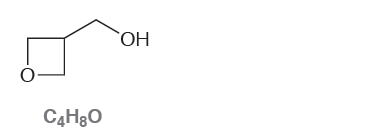# Problem: Consider the following compound with molecular formula  C 4H8O: (b) Draw a constitutional isomer that you expect will be less acidic than the compound above.

🤓 Based on our data, we think this question is relevant for Professor Baldwin's class at OSU.

###### Problem Details

Consider the following compound with molecular formula  C 4H8O:

(b) Draw a constitutional isomer that you expect will be less acidic than the compound above.What scientific concept do you need to know in order to solve this problem?

Our tutors have indicated that to solve this problem you will need to apply the pKa concept. You can view video lessons to learn pKa. Or if you need more pKa practice, you can also practice pKa practice problems.

What is the difficulty of this problem?

Our tutors rated the difficulty ofConsider the following compound with molecular formula  C 4H...as medium difficulty.

How long does this problem take to solve?

Our expert Organic tutor, Chris took undefined 52 seconds to solve this problem. You can follow their steps in the video explanation above.

What professor is this problem relevant for?

Based on our data, we think this problem is relevant for Professor Baldwin's class at OSU.

What textbook is this problem found in?

Our data indicates that this problem or a close variation was asked in Organic Chemistry - Klein 1st Edition. You can also practice Organic Chemistry - Klein 1st Edition practice problems.